Like   Tweet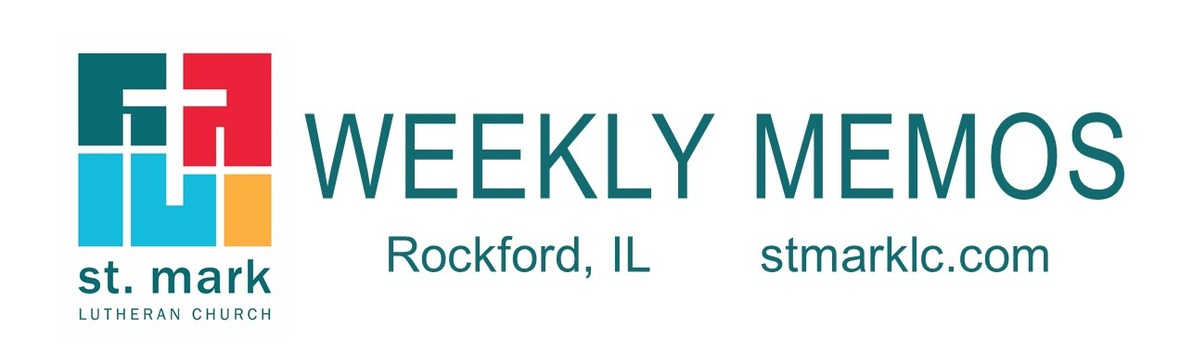table div table+table div table{width:100%;padding:0}table div table+table div table img{width:96.23%;padding:0;float:none}table div table+table div table td{width:100%;padding:0 1.88% 18px}/* styles */## Sunday, December 1 First Sunday of Advent

8:00 A.M. Worship
9:30 A.M. Worship
9:30 A.M. Sunday School
11:00 A.M. Worship

## Monday, December 2

9:00 A.M. Classic Chorale Rehearses
4:00 P.M. Deadline for MEMOS articles to be submitted
4:30 P.M. Yoga in the cafe
6:30 P.M. Emotions Anonymous

## Tuesday, December 3

9:00 A.M. Visitation for Tara Mufich
10:00 A.M. Memorial Service for Tara Mufich
11:00 A.M. St. Mark Duplicate Bridge
1:00 P.M. First Tuesday Book Club
2:00 P.M. Staff Meeting

## Wednesday, December 4

9:00 A.M. Women's Bible Study
9:00 A.M. Men's Bible Study
9:00 A.M. Small Bell Group
9:30 A.M. St. Mark Ringers
5:30 P.M. Good News Bearers
6:00 P.M. Bible Study
6:30 P.M. Confirmation
7:00 P.M. Voices of St. Mark

## Thursday, December 5

8:30 A.M. Primetimers Breakfast Meeting at Stockholm Inn
9:00 A.M. Cherry Valley Bridge Group
9:45 A.M. Primetimers to RRVP
6:00 P.M. Christmas Decorating Night
6:00 P.M. Diakonia
6:30 P.M. Blue Lights
6:30 P.M. Emotions Anonymous
7:00 P.M. Praise Band Rehearses

## Friday, December 6

7:30 A.M. St. Mark Men's Breakfast at Denny's

## Saturday, December 7

9:00 A.M. Concert Rehearsal

## Sunday, December 8 Second Sunday of Advent

8:00 A.M. Worship
9:30 A.M. Worship
9:30 A.M. Sunday School
11:00 A.M. Worship
3:00 P.M. Christmas at St. Mark Concert
7:00 P.M. 2nd Sunday at Katie's Cup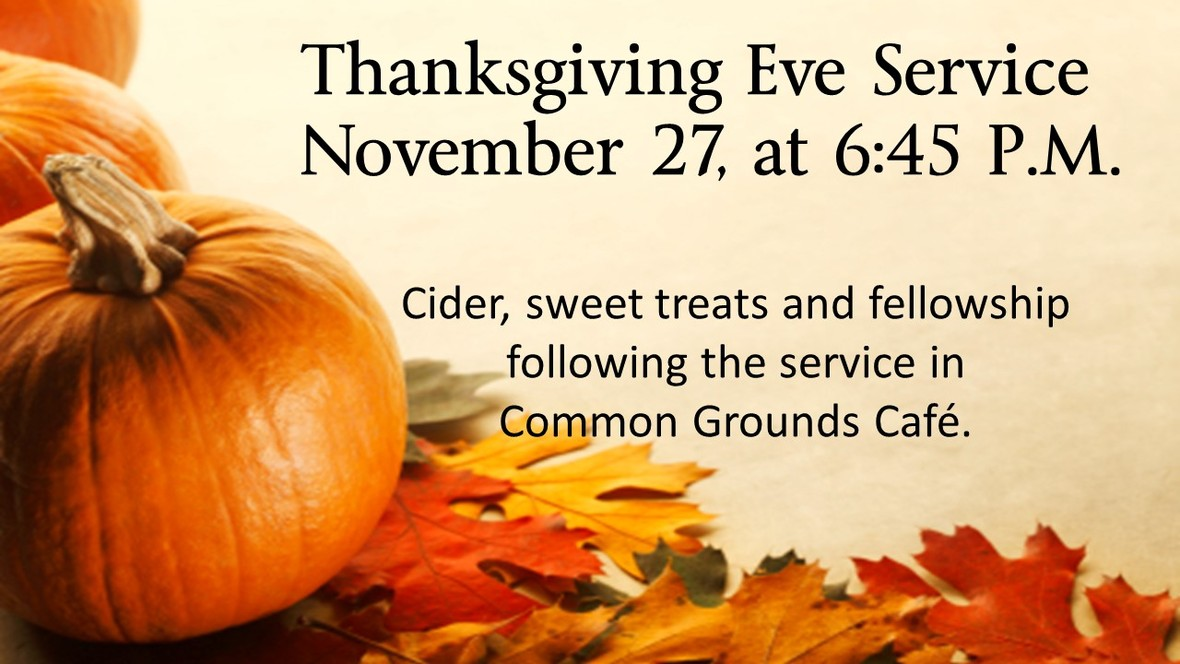table.module-6{width:84.91%;padding:0}table div table+table+table+table+table+table+table div table{width:84.91%;float:none;margin-left:auto;margin-right:auto;padding:0}table div table+table+table+table+table+table+table div table a{border:0 none;text-decoration:none}table div table+table+table+table+table+table+table div table img{width:100%!important;border:0 none;text-decoration:none}table div table+table+table+table+table+table+table div table td{width:100%;padding:0}/* styles */
 table div table+table+table+table+table+table+table+table div table{width:100%;padding:0}table div table+table+table+table+table+table+table+table div table img{width:96.23%;padding:0;float:none}table div table+table+table+table+table+table+table+table div table td{width:100%;padding:0 1.88% 18px}/* styles */## News and Events

 table div table+table+table+table+table+table+table+table+table+table div table{width:100%;padding:0}table div table+table+table+table+table+table+table+table+table+table div table img{width:96.23%;padding:0;float:none}table div table+table+table+table+table+table+table+table+table+table div table td{width:100%;padding:0 1.88% 18px}/* styles */Pledge Update
On October 20, we announced our 2010 Congregation Budget goal of \$827,281.00, a small increase of only \$1,481 over our 2019 budget. To date, we have received 242 pledges, totaling \$719,794.00 This amount represents 96% of the \$750,751.00 of pledged giving needed for 2020. Our pledged giving of \$750,751.00 is a key part of our total budget of \$827,281.00. This year’s stewardship campaign will continue each week until our congregation budget goal is reached. We thank you for your participation in this important expression of support and gratitude. Together, we can keep our ministry moving forward with confidence and hope.

If you have not turned in your pledge card for 2020, please do so. With everyone's assistance, we can better anticipate the full level of support from all our members for the coming year.

 table div table+table+table+table+table+table+table+table+table+table+table+table div table{width:100%;padding:0}table div table+table+table+table+table+table+table+table+table+table+table+table div table img{width:96.23%;padding:0;float:none}table div table+table+table+table+table+table+table+table+table+table+table+table div table td{width:100%;padding:0 1.88% 18px}/* styles */All Circle Christmas Lunch
The St. Mark Women’s Circles will gather at Tavern on Clark on December 12, at 11:30 for a combined Christmas Luncheon. Guests are always welcome. Please call your Circle Leader to RSVP. Contact Judy Penticoff if you need further information. teachem4@comcast.net or 815 985-3427

 table div table+table+table+table+table+table+table+table+table+table+table+table+table+table div table{width:100%;padding:0}table div table+table+table+table+table+table+table+table+table+table+table+table+table+table div table img{width:96.23%;padding:0;float:none}table div table+table+table+table+table+table+table+table+table+table+table+table+table+table div table td{width:100%;padding:0 1.88% 18px}/* styles */St. Mark Mobile App!
This is different than our church directory app.
Our mobile app is designed specifically for St. Mark.

It will improve our communication with our congregation and nurture our members. A mobile app is important in the digital age. Never wonder how to get involved at St. Mark.

You will use this app for everything! You will be able to sign up for events, stay connected to your ministry group, give, send prayer requests, have an updated calendar, learn how to get involved and so much for.

We encourage you to download this app. The St. Mark app will be such a viable asset for you. It's about getting involved and staying connected.

If you have any questions, please call the office at 815-398-3557 or email Jill at Jill@stmarklc.com.

 table div table+table+table+table+table+table+table+table+table+table+table+table+table+table+table+table div table{width:100%;padding:0}table div table+table+table+table+table+table+table+table+table+table+table+table+table+table+table+table div table img{width:96.23%;padding:0;float:none}table div table+table+table+table+table+table+table+table+table+table+table+table+table+table+table+table div table td{width:100%;padding:0 1.88% 18px}/* styles */Place your order for a poinsettia

We will be decorating the altar with beautiful white and red poinsettias again this Christmas Eve. There are order forms in the narthex and you can pay using our new mobile app or with cash or check. The plants are \$10.00 each. This is a wonderful way to honor or remember someone you love this holiday season. You will be able to take your plant home after the Christmas Eve services.

 table div table+table+table+table+table+table+table+table+table+table+table+table+table+table+table+table+table+table div table{width:100%;padding:0}table div table+table+table+table+table+table+table+table+table+table+table+table+table+table+table+table+table+table div table img{width:96.23%;padding:0;float:none}table div table+table+table+table+table+table+table+table+table+table+table+table+table+table+table+table+table+table div table td{width:100%;padding:0 1.88% 18px}/* styles */Wassail Sunday

 table div table+table+table+table+table+table+table+table+table+table+table+table+table+table+table+table+table+table+table+table div table{width:100%;padding:0}table div table+table+table+table+table+table+table+table+table+table+table+table+table+table+table+table+table+table+table+table div table img{width:96.23%;padding:0;float:none}table div table+table+table+table+table+table+table+table+table+table+table+table+table+table+table+table+table+table+table+table div table td{width:100%;padding:0 1.88% 18px}/* styles */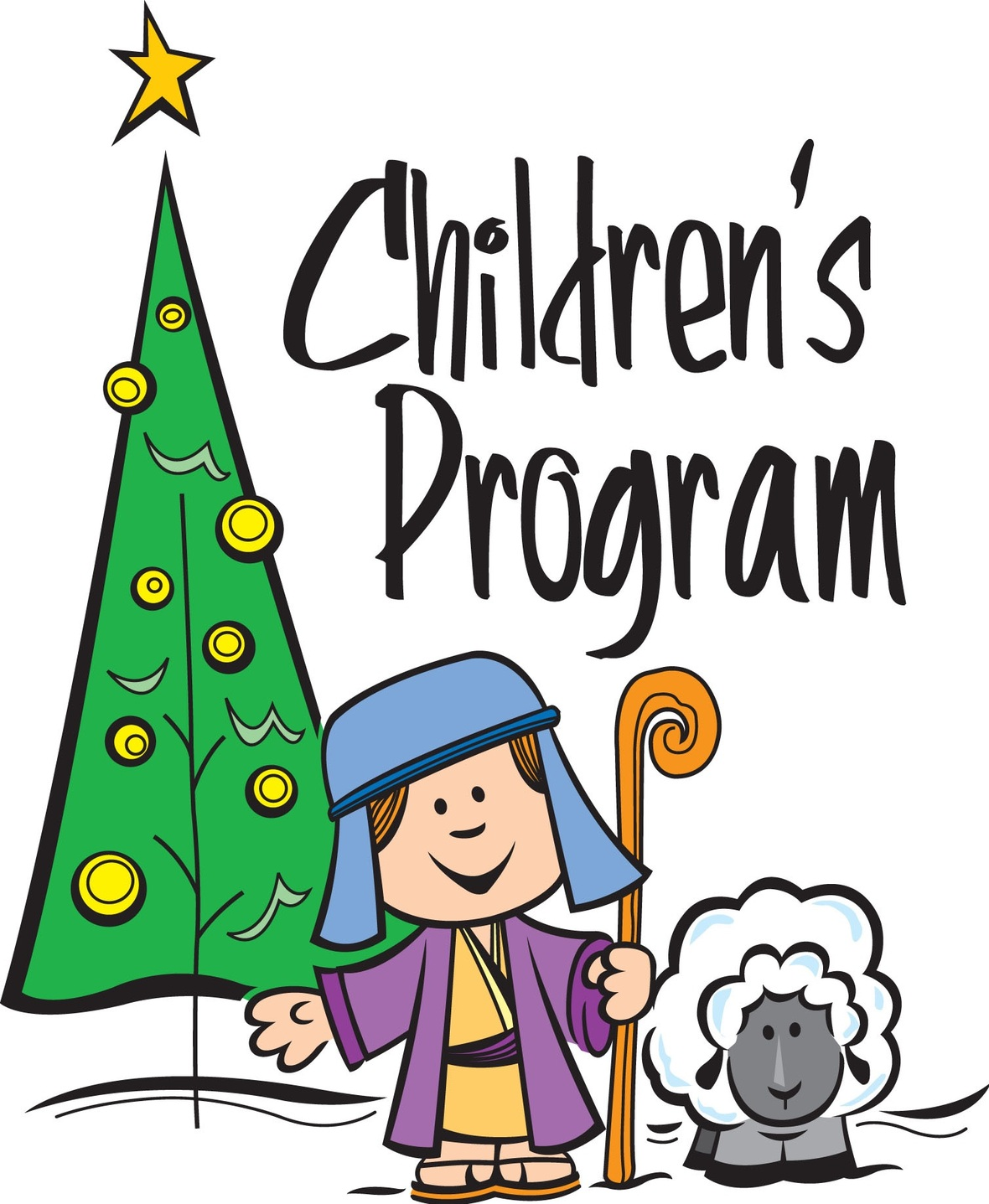Hear ye, hear ye!
Hear ye, hear ye! Bethlehem Court is now in session, and is about to hear The Case of The Reluctant Innkeeper! What exactly happened that night, when Joseph and Mary arrived and asked for a room at the inn? We’ll hear from several witnesses and even the innkeeper himself, and the jury will do their best to decide if he violated Bethlehem Code Number 982 when he put them in the stable.

Join the Sunday School Singers and Good News Bearers at the 9:30 service on December 15 for this fun Christmas program!

 table div table+table+table+table+table+table+table+table+table+table+table+table+table+table+table+table+table+table+table+table+table+table div table{width:100%;padding:0}table div table+table+table+table+table+table+table+table+table+table+table+table+table+table+table+table+table+table+table+table+table+table div table img{width:96.23%;padding:0;float:none}table div table+table+table+table+table+table+table+table+table+table+table+table+table+table+table+table+table+table+table+table+table+table div table td{width:100%;padding:0 1.88% 18px}/* styles */Request for Nursery Volunteers
There is still a need for volunteers to assist the nursery staff from 9:15 – 10:45 on Sunday mornings. Please follow this link
to sign up for one or more of the listed dates. The nursery is a valuable part of our hospitality ministry to the families and visitors of St. Mark who have young children. It is also one of the most fun ways to serve our community!

Most notably, we're in need of volunteers for December 8 & 15.

Volunteers are expected to assist Libby or Anya - our nursery staff - in caring for the children ages 6 mo – 3 years who are in the nursery during the 9:30 service. This includes playtime, other activities such as reading or art, helping with snacks, and cleanup. Staff are responsible for diaper changes, etc.

Adults and high school youth are invited to volunteer – and youth, you can get service hours for your time in the nursery!

 table div table+table+table+table+table+table+table+table+table+table+table+table+table+table+table+table+table+table+table+table+table+table+table+table div table{width:100%;padding:0}table div table+table+table+table+table+table+table+table+table+table+table+table+table+table+table+table+table+table+table+table+table+table+table+table div table img{width:96.23%;padding:0;float:none}table div table+table+table+table+table+table+table+table+table+table+table+table+table+table+table+table+table+table+table+table+table+table+table+table div table td{width:100%;padding:0 1.88% 18px}/* styles */We will decorate the church for Christmas on Thursday, December 5 beginning at 6:00 P.M. Folks can report to Fellowship Hall to help, and all are welcome.

 table div table+table+table+table+table+table+table+table+table+table+table+table+table+table+table+table+table+table+table+table+table+table+table+table+table+table div table{width:100%;padding:0}table div table+table+table+table+table+table+table+table+table+table+table+table+table+table+table+table+table+table+table+table+table+table+table+table+table+table div table img{width:96.23%;padding:0;float:none}table div table+table+table+table+table+table+table+table+table+table+table+table+table+table+table+table+table+table+table+table+table+table+table+table+table+table div table td{width:100%;padding:0 1.88% 18px}/* styles */Advent begins on Sunday, December 1. There are devotional booklets for you to pick up on the tables before you enter the Sanctuary. I Wonder As I Wander, by Michelle Van Loon will offer you a relevant Bible passage on which to reflect, a meaningful meditation, a short prayer and a suggestion for additional Scripture readings in order to guide you on this journey.

 table div table+table+table+table+table+table+table+table+table+table+table+table+table+table+table+table+table+table+table+table+table+table+table+table+table+table+table+table div table{width:100%;padding:0}table div table+table+table+table+table+table+table+table+table+table+table+table+table+table+table+table+table+table+table+table+table+table+table+table+table+table+table+table div table img{width:96.23%;padding:0;float:none}table div table+table+table+table+table+table+table+table+table+table+table+table+table+table+table+table+table+table+table+table+table+table+table+table+table+table+table+table div table td{width:100%;padding:0 1.88% 18px}/* styles */Yoga is Happening at St. Mark!
Join us on December 2 and 9 at 4:30 p.m. in the St. Mark Café space for Yoga. Dress comfortable, if you have a yoga mat bring it…otherwise, we have mats you may borrow. Also, bring a water bottle. Betsy Hornick is our yoga instructor and she is doing a great job of leading us through the workout.

 table div table+table+table+table+table+table+table+table+table+table+table+table+table+table+table+table+table+table+table+table+table+table+table+table+table+table+table+table+table+table div table{width:100%;padding:0}table div table+table+table+table+table+table+table+table+table+table+table+table+table+table+table+table+table+table+table+table+table+table+table+table+table+table+table+table+table+table div table img{width:96.23%;padding:0;float:none}table div table+table+table+table+table+table+table+table+table+table+table+table+table+table+table+table+table+table+table+table+table+table+table+table+table+table+table+table+table+table div table td{width:100%;padding:0 1.88% 18px}/* styles */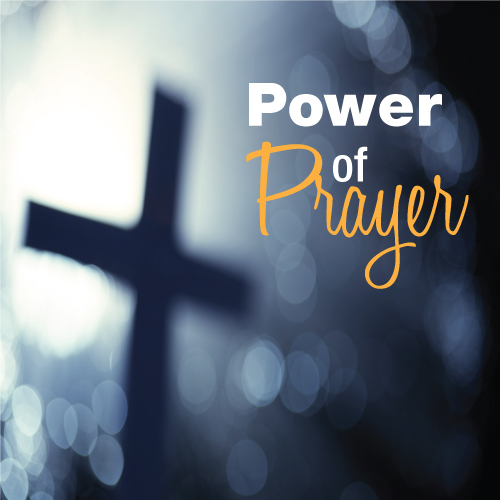St. Mark Prayer Chain
Would you like to be a part of the St. Mark prayer chain? Prayer requests come into the church office weekly. As we receive prayer requests we would send out an e-mail and ask that you pray daily for the persons mentioned in the requests. All requests are to remain confidential. Please e-mail melinda@stmarklc.com if you would like to participate in the prayer chain. “Be anxious for nothing, but in everything by prayer and supplication, with thanksgiving, let your requests be made known to God.” Colossians 4:2.

 table div table+table+table+table+table+table+table+table+table+table+table+table+table+table+table+table+table+table+table+table+table+table+table+table+table+table+table+table+table+table+table+table div table{width:100%;padding:0}table div table+table+table+table+table+table+table+table+table+table+table+table+table+table+table+table+table+table+table+table+table+table+table+table+table+table+table+table+table+table+table+table div table img{width:96.23%;padding:0;float:none}table div table+table+table+table+table+table+table+table+table+table+table+table+table+table+table+table+table+table+table+table+table+table+table+table+table+table+table+table+table+table+table+table div table td{width:100%;padding:0 1.88% 18px}/* styles */Instant Church Directory
If you have not submitted a family photo for the online directory, please follow the links below.
It is simple and takes only a few minutes to upload your photo and then download the Instant Church Directory App. You will always have updated members' information at your fingertips.

Instant Church Directory

Once you have downloaded the app and created an account, you are not able to change or update your information, the system administrator is the only one to change and update the information. If something is incorrect, please contact the church office at 815-398-3557 or email jill@stmarklc.com.

Mobile App Login Instructions for Android & Kindle Devices

We also have a form for you to complete to make sure we have the most current information for the directory. There are paper forms at church or click on the link to Church Directory Form

 table div table+table+table+table+table+table+table+table+table+table+table+table+table+table+table+table+table+table+table+table+table+table+table+table+table+table+table+table+table+table+table+table+table+table div table{width:100%;padding:0}table div table+table+table+table+table+table+table+table+table+table+table+table+table+table+table+table+table+table+table+table+table+table+table+table+table+table+table+table+table+table+table+table+table+table div table img{width:96.23%;padding:0;float:none}table div table+table+table+table+table+table+table+table+table+table+table+table+table+table+table+table+table+table+table+table+table+table+table+table+table+table+table+table+table+table+table+table+table+table div table td{width:100%;padding:0 1.88% 18px}/* styles */The 2020 altar flower chart is up in Fellowship Hall on the cabinets next to the coffee station on Sunday mornings. The arrangements will be \$35. This is a great way to honor or remember that special
someone or that special anniversary.

 table div table+table+table+table+table+table+table+table+table+table+table+table+table+table+table+table+table+table+table+table+table+table+table+table+table+table+table+table+table+table+table+table+table+table+table+table div table{width:100%;padding:0}table div table+table+table+table+table+table+table+table+table+table+table+table+table+table+table+table+table+table+table+table+table+table+table+table+table+table+table+table+table+table+table+table+table+table+table+table div table img{width:96.23%;padding:0;float:none}table div table+table+table+table+table+table+table+table+table+table+table+table+table+table+table+table+table+table+table+table+table+table+table+table+table+table+table+table+table+table+table+table+table+table+table+table div table td{width:100%;padding:0 1.88% 18px}/* styles */The First Tuesday Book Club
The next meeting is at 1:00 pm on December 3 at the home of Jane Lundstrom. Please email Jane at jrichlun@gmail.com and let her know if you will attend, or leave a message at (815) 226-8725.

ALL are welcome. We’ll read and discuss Fruit of the Drunken Tree by Ingrid Rojas Contreras. Sandy Lindstrom will be the reviewer and discussion leader. The novel explores a complex and destructive friendship against the background of Columbia’s political violence. Looking ahead, our January book is The Year of Pleasures by Elizabeth Berg.

 table div table+table+table+table+table+table+table+table+table+table+table+table+table+table+table+table+table+table+table+table+table+table+table+table+table+table+table+table+table+table+table+table+table+table+table+table+table+table div table{width:100%;padding:0}table div table+table+table+table+table+table+table+table+table+table+table+table+table+table+table+table+table+table+table+table+table+table+table+table+table+table+table+table+table+table+table+table+table+table+table+table+table+table div table img{width:96.23%;padding:0;float:none}table div table+table+table+table+table+table+table+table+table+table+table+table+table+table+table+table+table+table+table+table+table+table+table+table+table+table+table+table+table+table+table+table+table+table+table+table+table+table div table td{width:100%;padding:0 1.88% 18px}/* styles */## Youth Ministry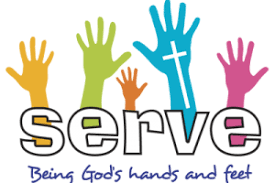Service Learning Camps Summer 2020!
“Where the learning is just as important as the service.” If you will be entering high school in the fall of 2020 or are already in high school then this is for you! We will be traveling to Toledo, Ohio July 12-17th, 2020. There will be service projects and a day of sightseeing.

We need to get a commitment by January 1, if you are interested. The cost is \$350, with a deposit of \$50. Due January 1, 2020.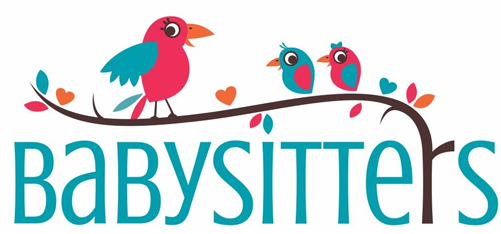Middle School and High School Youth “babysitter” list.
On occasion, parents will ask for the name of one of our youth who babysits.
Would you like to be on that list? You don’t have to be a certified babysitter, but if you take a class or course let me know. Please e-mail melinda@stmarklc.com or text me at 815-871-0390. Need more information see Melinda Alekna.

 table div table+table+table+table+table+table+table+table+table+table+table+table+table+table+table+table+table+table+table+table+table+table+table+table+table+table+table+table+table+table+table+table+table+table+table+table+table+table+table+table+table+table div table{width:100%;padding:0}table div table+table+table+table+table+table+table+table+table+table+table+table+table+table+table+table+table+table+table+table+table+table+table+table+table+table+table+table+table+table+table+table+table+table+table+table+table+table+table+table+table+table div table img{width:96.23%;padding:0;float:none}table div table+table+table+table+table+table+table+table+table+table+table+table+table+table+table+table+table+table+table+table+table+table+table+table+table+table+table+table+table+table+table+table+table+table+table+table+table+table+table+table+table+table div table td{width:100%;padding:0 1.88% 18px}/* styles */## Outreach MinistryFoster children gifts must be turned in to the Red Truck by Sunday, December 1st. We have had a wonderful response from St. Mark members who took Angel Tags and shopped for a gift for their child. Lutheran Social Services representative Bob Coffey will be coming to the church at 11:00 AM on Monday, December 2nd to pick up the gifts. Thanks to all who participated in this heartwarming project. The Outreach Committee is very grateful for your support.

 table div table+table+table+table+table+table+table+table+table+table+table+table+table+table+table+table+table+table+table+table+table+table+table+table+table+table+table+table+table+table+table+table+table+table+table+table+table+table+table+table+table+table+table+table+table div table{width:100%;padding:0}table div table+table+table+table+table+table+table+table+table+table+table+table+table+table+table+table+table+table+table+table+table+table+table+table+table+table+table+table+table+table+table+table+table+table+table+table+table+table+table+table+table+table+table+table+table div table img{width:96.23%;padding:0;float:none}table div table+table+table+table+table+table+table+table+table+table+table+table+table+table+table+table+table+table+table+table+table+table+table+table+table+table+table+table+table+table+table+table+table+table+table+table+table+table+table+table+table+table+table+table+table div table td{width:100%;padding:0 1.88% 18px}/* styles */## Primetimers Ministry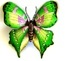December 5, THURSDAY - Breakfast meeting at the Stockholm Restaurant.

December 14, SATURDAY - 9:30 a.m. Meet at church to set up and decorate for Wassail Sunday.

 table div table+table+table+table+table+table+table+table+table+table+table+table+table+table+table+table+table+table+table+table+table+table+table+table+table+table+table+table+table+table+table+table+table+table+table+table+table+table+table+table+table+table+table+table+table+table+table+table div table{width:100%;padding:0}table div table+table+table+table+table+table+table+table+table+table+table+table+table+table+table+table+table+table+table+table+table+table+table+table+table+table+table+table+table+table+table+table+table+table+table+table+table+table+table+table+table+table+table+table+table+table+table+table div table img{width:96.23%;padding:0;float:none}table div table+table+table+table+table+table+table+table+table+table+table+table+table+table+table+table+table+table+table+table+table+table+table+table+table+table+table+table+table+table+table+table+table+table+table+table+table+table+table+table+table+table+table+table+table+table+table+table div table td{width:100%;padding:0 1.88% 18px}/* styles */table div table+table+table+table+table+table+table+table+table+table+table+table+table+table+table+table+table+table+table+table+table+table+table+table+table+table+table+table+table+table+table+table+table+table+table+table+table+table+table+table+table+table+table+table+table+table+table+table+table+table div table{width:100%;padding:0}table div table+table+table+table+table+table+table+table+table+table+table+table+table+table+table+table+table+table+table+table+table+table+table+table+table+table+table+table+table+table+table+table+table+table+table+table+table+table+table+table+table+table+table+table+table+table+table+table+table+table div table img{width:96.23%;padding:0;float:none}table div table+table+table+table+table+table+table+table+table+table+table+table+table+table+table+table+table+table+table+table+table+table+table+table+table+table+table+table+table+table+table+table+table+table+table+table+table+table+table+table+table+table+table+table+table+table+table+table+table+table div table td{width:100%;padding:0 1.88% 18px}/* styles */## Our St. Mark members:

Bette Patterson, Jason Carlson, Beverly Wright, Jim Tooley, Jerry Cazaniga, David Young, Keith Haggestad.

## Our condolences to:

Chuck Mufich and family upon the death of his wife, Tara.

Patt Schmoldt upon the death of her sister, Joyce.

## Family and friends of St. Mark members:

Mike Montalbano, Anne Olson, Jessica Salter, Bill Ganschow, Tim Babcock, Polly Young, Eva Love Sherbondy, Matthew and David Lundine, Jennifer Peterson, Dale Tallmadge, Pastor Jane McChesney, Earl Karau, Clay Heinrich, Denise Nichols, Mary Graham, Kim Menke, Norma Comstock, Ethan Whitney, Carolyn King, Katie Butera, Ashton Henley, Nathan Gyllin.

## Those serving in the military and their families:

Tristan Davenport, Scot Hornick, Bailey Panjkovich, Xander Gehrke, Jim Tammen, Brandon Ballenger, Taylor Eversole, Dean Barron.

 table div table+table+table+table+table+table+table+table+table+table+table+table+table+table+table+table+table+table+table+table+table+table+table+table+table+table+table+table+table+table+table+table+table+table+table+table+table+table+table+table+table+table+table+table+table+table+table+table+table+table+table+table+table div table{width:100%;padding:0}table div table+table+table+table+table+table+table+table+table+table+table+table+table+table+table+table+table+table+table+table+table+table+table+table+table+table+table+table+table+table+table+table+table+table+table+table+table+table+table+table+table+table+table+table+table+table+table+table+table+table+table+table+table div table img{width:96.23%;padding:0;float:none}table div table+table+table+table+table+table+table+table+table+table+table+table+table+table+table+table+table+table+table+table+table+table+table+table+table+table+table+table+table+table+table+table+table+table+table+table+table+table+table+table+table+table+table+table+table+table+table+table+table+table+table+table+table div table td{width:100%;padding:0 1.88% 18px}/* styles *//* styles */ Office Hours Monday - Friday, 8:00 A.M. - 4:00 P.M. Telephone: 815-398-3557 Publications St. Mark Memos, the weekly e-newsletter, is distributed each Thursday. The deadline for submission is Monday by 4:00 P.M. Please click to send submissions. Sermons Online Miss a Sunday at St. Mark? Please visit our website and watch the sermons online. Please click to watch.